Logarithms identities

Logarithm of b to base a is called the power which the a should be exponentiated to get the b:

c = log a bac = b , and b > 0, a > 0, a1

a log a b = b
log a 1 = 0
log a a = 1
log a an = n

Logarithm of the product:

log a (uv) = log a u + log a v

Logarithm of the division: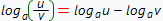Logarithm of the powers and roots:

log a u n = nlog a u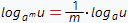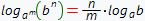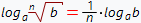Transfer to the new logarithm base formula: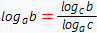Formulas, derived from logarithm features: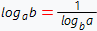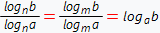log n alog m b = log m alog n b
a log n b = b log n a

Logarithms comparison: